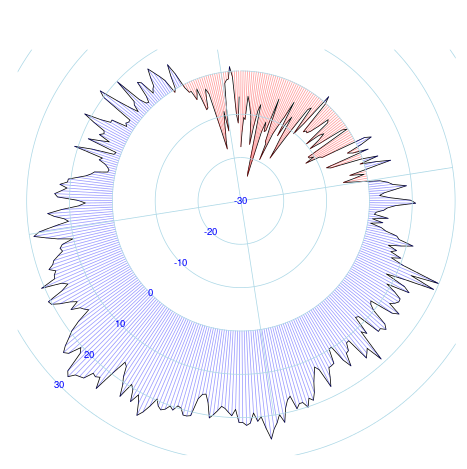{{announcement.body}}
{{announcement.title}}

# Radial Graphs for Time Series

DZone 's Guide to

# Radial Graphs for Time Series

### A code snippet in Mathmatica to produce a radial graph to visualize a range of temperatures.

· Big Data Zone ·
Free Resource

Comment (0)

Save
{{ articles.views | formatCount}} Views

On How to: Weather Radials, there was a nice visualization of temperatures. Since I am too old fashioned for ggplot2, I wanted to reproduce a similar graph with the old plot style.

Assume that daily temperature is in a vector X (e.g. temperature in Montréal, QC, in 2009). To get a radial plot, use:

``````> n=length(X)
> theta=seq(0,1-1/n,length=n)*2*pi
> r=30+X
> plot(r*cos(pi/2-theta),r*sin(pi/2-theta),type="l",xlab="",ylab="",axes=FALSE)
> for(t in 1:n){
+   if(X[t]>0) CL=rgb(0,0,1,.4)
+   if(X[t]<0) CL=rgb(1,0,0,.4)
+   if(X[t]==0) CL="white"
+   segments((30+X[t])*cos(pi/2-theta[t]),(30+X[t])*sin(pi/2-theta[t]),30*cos(pi/2-theta[t]),30*sin(pi/2-theta[t]),col=CL)
+ }
> for(r in 10*seq(0,6)) lines(r*cos(pi/2-theta),r*sin(pi/2-theta),type="l",col="light blue")``````Topics:

Comment (0)

Save
{{ articles.views | formatCount}} Views

Published at DZone with permission of Arthur Charpentier , DZone MVB. See the original article here.

Opinions expressed by DZone contributors are their own.